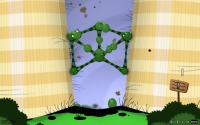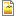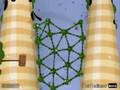# Ivy Towers

### Ivy Towers

#### The Goo Filled HillsNormal: 2 balls
OCD: 16 balls

Previous level:
Impale Sticky.
Next level:
Tumbler.

### brace yourself

Use the Ivy Goo Balls to climb up by removing the ones at the bottom and placing them at the top.

#### Signposts

A new species of GooBall!
I must say, they look quite beautiful...
Maybe they have commitment anxiety, I'm not sure,
but it looks like they can be easily detached from each other

If a Goo Ball falls into a well 10 meters deep...
and climbs up 3 meters each day...
but slides down 2 meters at night...
will he still look fabulous in the morning?
...maybe if he were to brace against the walls as hard as he could, and believe in the power of friction...
...he could leave the ground behind...

brace yourself
Level data
Directory name:
IvyTower
Island:
Chapter 1 (The Goo Filled Hills)
Official:
Yes
Normal Requirement:
2
OCD Type:
Balls
OCD Requirement:
16
Depends on:
Screenshots:Untitled-1.png
Videos:
You need Flash player 8+ and JavaScript enabled to view this video.World of Goo OCD - Ivy Towers
by davidc32346.
Rating: 0.00.

There is 1 video.

Balls Used

• 17 Ivy (1 sleeping, 6 attached)
```<level ballsrequired="2" letterboxed="false" visualdebug="false" autobounds="false" textcolor="255,255,255" timebugprobability="0.333" strandgeom="false" >

<!-- Camera -->
<camera aspect="normal" endpos="0,932.01" endzoom="1.002">
<poi pos="0,1000" traveltime="0" pause="0" zoom="1" />
<poi pos="0,306.01" traveltime="2" pause="1" zoom="1" />
</camera>
<camera aspect="widescreen" endpos="0,932.01" endzoom="1.334">
<poi pos="0,1070" traveltime="0" pause="0" zoom="1.334" />
<poi pos="0,231.01" traveltime="2" pause="1" zoom="1.334" />
</camera>

<!-- Music -->
<music id="SOUND_LEVEL_IVYTOWER_TEMP_DWTD2" />

<!-- Fire -->

<!-- Signposts -->
<signpost name="signpost_brown" depth="40" x="-237.44" y="762.99" scalex="0.817" scaley="0.817" rotation="-91.32" alpha="1" colorize="255,255,255" image="IMAGE_SCENE_IVYTOWER_SIGNPOST_BROWN" text="SIGNPOST_IVYTOWER_2"   />
<signpost name="signpost_brown" depth="40" x="269.7" y="150.65" scalex="0.817" scaley="0.817" rotation="-7.91" alpha="1" colorize="255,255,255" image="IMAGE_SCENE_IVYTOWER_SIGNPOST_BROWN" text="SIGNPOST_IVYTOWER_1"   />

<!-- Pipes -->
<pipe id="0" depth="-12" >
<Vertex x="0" y="1175" />
<Vertex x="0" y="1250" />
<Vertex x="275" y="1250" />
</pipe>

<!-- Balls -->
<BallInstance type="Ivy" x="116" y="902" id="0" angle="0" />
<BallInstance type="Ivy" x="23" y="86" id="1" angle="0" />
<BallInstance type="Ivy" x="0" y="123" id="2" angle="0" />
<BallInstance type="Ivy" x="53" y="2349" id="3" angle="0" />
<BallInstance type="Ivy" x="-42" y="99" id="4" angle="0" />
<BallInstance type="Ivy" x="-124" y="1825" id="5" angle="0" />
<BallInstance type="Ivy" x="-50" y="2601" id="6" angle="0" />
<BallInstance type="Ivy" x="63" y="1073" id="7" angle="0" />
<BallInstance type="Ivy" x="84" y="1396" id="8" angle="0" />
<BallInstance type="Ivy" x="-136" y="1265" id="9" angle="0" />
<BallInstance type="Ivy" x="136" y="1130.49" id="10" angle="0" />
<BallInstance type="Ivy" x="-1" y="252.99" id="11" angle="0" />
<BallInstance type="Ivy" x="0" y="351.99" id="12" angle="0" />
<BallInstance type="Ivy" x="88" y="210.5" id="13" angle="0" />
<BallInstance type="Ivy" x="-92.99" y="210.5" id="14" angle="0" />
<BallInstance type="Ivy" x="-104.49" y="332.5" id="15" angle="0" />
<BallInstance type="Ivy" x="99.5" y="334.5" id="16" angle="0" />

<!-- Arms -->
<Strand gb1="11" gb2="13" />
<Strand gb1="12" gb2="11" />
<Strand gb1="16" gb2="12" />
<Strand gb1="11" gb2="14" />
<Strand gb1="15" gb2="14" />
<Strand gb1="12" gb2="15" />
<Strand gb1="13" gb2="16" />
<Strand gb1="16" gb2="11" />
<Strand gb1="15" gb2="11" />

<!-- Level Exit -->
<levelexit id="theExit" pos="0,1175" radius="75" filter="" >
</levelexit>

</level>```
```<scene minx="-400" miny="0" maxx="400" maxy="1300" backgroundcolor="0,0,0" >

<!-- ForceFields -->
<linearforcefield type="gravity" force="0,-10" dampeningfactor="0" antigrav="true" />

<!-- Particles -->
<particles effect="rainingleaves" depth="-80" pretick="0" />

<!-- SceneLayers -->
<SceneLayer name="bush_leafy_1" depth="160" x="278.48" y="37.01" scalex="1.25" scaley="1.25" rotation="19.98" alpha="1" colorize="255,255,255" image="IMAGE_SCENE_IVYTOWER_BUSH_LEAFY_1"   />
<SceneLayer name="bush_leafy_1" depth="160" x="-261.53" y="15.01" scalex="1.25" scaley="1.25" rotation="0" alpha="1" colorize="255,255,255" image="IMAGE_SCENE_IVYTOWER_BUSH_LEAFY_1"   />
<SceneLayer name="bush_leafy_1b" depth="80" x="274.42" y="68.8" scalex="1" scaley="1" rotation="26.94" alpha="1" colorize="255,255,255" image="IMAGE_SCENE_IVYTOWER_BUSH_LEAFY_1B"   />
<SceneLayer name="bush_leafy_1b" depth="80" x="-278.52" y="57.01" scalex="1" scaley="1" rotation="-18.05" alpha="1" colorize="255,255,255" image="IMAGE_SCENE_IVYTOWER_BUSH_LEAFY_1B"   />
<SceneLayer name="bush_leafy_1" depth="48" x="-389.82" y="773.27" scalex="0.811" scaley="0.811" rotation="-79.55" alpha="1" colorize="255,255,255" image="IMAGE_SCENE_IVYTOWER_BUSH_LEAFY_1"   />
<SceneLayer name="signpostPole_brown" depth="39.2" x="-295.53" y="761.29" scalex="0.833" scaley="1.867" rotation="-88.05" alpha="1" colorize="255,255,255" image="IMAGE_SCENE_IVYTOWER_SIGNPOSTPOLE_BROWN"   />
<SceneLayer name="signpostPole_brown" depth="39.2" x="268.46" y="111.18" scalex="0.833" scaley="1.403" rotation="3.1" alpha="1" colorize="255,255,255" image="IMAGE_SCENE_IVYTOWER_SIGNPOSTPOLE_BROWN"   />
<SceneLayer name="ground" depth="0.8" x="0" y="59.5" scalex="1" scaley="1" rotation="0" alpha="1" colorize="255,255,255" image="IMAGE_SCENE_IVYTOWER_GROUND"   />
<SceneLayer name="TowerLeft" depth="0" x="-244.58" y="650.04" scalex="1" scaley="1.27" rotation="0" alpha="1" colorize="255,255,255" image="IMAGE_SCENE_IVYTOWER_TOWERLEFT"   />
<SceneLayer name="TowerRight" depth="0" x="238.42" y="650.04" scalex="1" scaley="1.27" rotation="0" alpha="1" colorize="255,255,255" image="IMAGE_SCENE_IVYTOWER_TOWERRIGHT"   />
<SceneLayer name="grassBlack" depth="-8" x="-147.64" y="891.52" scalex="1" scaley="0.697" rotation="-81.15" alpha="1" colorize="255,255,255" image="IMAGE_SCENE_IVYTOWER_GRASSBLACK"   />
<SceneLayer name="grassBlack" depth="-8" x="-95.15" y="249.55" scalex="1" scaley="0.697" rotation="-81.15" alpha="1" colorize="255,255,255" image="IMAGE_SCENE_IVYTOWER_GRASSBLACK"   />
<SceneLayer name="grassBlack" depth="-8" x="-129.64" y="543.54" scalex="1" scaley="0.697" rotation="-81.15" alpha="1" colorize="255,255,255" image="IMAGE_SCENE_IVYTOWER_GRASSBLACK"   />
<SceneLayer name="grassBlack" depth="-8" x="182.33" y="1070.03" scalex="1" scaley="0.697" rotation="83.85" alpha="1" colorize="255,255,255" image="IMAGE_SCENE_IVYTOWER_GRASSBLACK"   />
<SceneLayer name="grassBlack" depth="-8" x="150.84" y="717.55" scalex="1" scaley="0.697" rotation="83.85" alpha="1" colorize="255,255,255" image="IMAGE_SCENE_IVYTOWER_GRASSBLACK"   />
<SceneLayer name="grassBlack" depth="-8" x="111.84" y="347.07" scalex="1" scaley="0.697" rotation="83.85" alpha="1" colorize="255,255,255" image="IMAGE_SCENE_IVYTOWER_GRASSBLACK"   />
<SceneLayer name="bush_leafy_1" depth="-40" x="-213.82" y="1150.26" scalex="0.811" scaley="0.811" rotation="-69.07" alpha="1" colorize="255,255,255" image="IMAGE_SCENE_IVYTOWER_BUSH_LEAFY_1"   />
<SceneLayer name="bush_leafy_1" depth="-40" x="353.18" y="1077.26" scalex="0.811" scaley="0.811" rotation="-65.51" alpha="1" colorize="255,255,255" image="IMAGE_SCENE_IVYTOWER_BUSH_LEAFY_1"   />
<SceneLayer name="bush_leafy_1" depth="-40" x="-368.81" y="1071.26" scalex="0.811" scaley="0.811" rotation="99.84" alpha="1" colorize="255,255,255" image="IMAGE_SCENE_IVYTOWER_BUSH_LEAFY_1"   />
<SceneLayer name="bush_leafy_1" depth="-40" x="221.19" y="1219.26" scalex="0.811" scaley="0.811" rotation="99.84" alpha="1" colorize="255,255,255" image="IMAGE_SCENE_IVYTOWER_BUSH_LEAFY_1"   />
<SceneLayer name="bg" depth="-640" x="0.01" y="623.4" scalex="1.563" scaley="2.006" rotation="0" alpha="1" colorize="255,255,255" image="IMAGE_SCENE_IVYTOWER_BG"   />

<!-- Buttons -->

<!-- Labels -->

<!-- Static Geometry -->
<line id="left" static="true" tag="unwalkable" material="IvyTower" anchor="-147,671" normal="0.9962,0.0876" />
<line id="right" static="true" tag="unwalkable" material="IvyTower" anchor="151,697" normal="-0.9947,0.1026" />
<line id="" static="true" material="rock" anchor="-69,64" normal="-0.002,1" />

<!-- Dynamic Geometry -->

<!-- Geometry Constraints -->
</scene>```